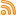#Put Call Ratio

## How To Interpret Put-Call Ratios

April 24, 2012If you’re a new option trader, knowing the basic idea of how to read put-to-call ratios can be a valuable piece of information. The ratio acts as an indicator by simply looking at the day’s put volume divided by call volume.  Since the ratio is calculated by dividing the number of puts by calls, the […]